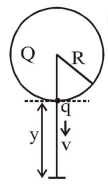# A solid sphere of radius $R$ carries a charge

Question:

A solid sphere of radius $R$ carries a charge $(\mathrm{Q}+\mathrm{q})$ distributed uniformly over its volume. A very small point like piece of it of mass $m$ gets detached from the bottom of the sphere and falls down vertically under gravity. This piece carries charge $\mathrm{q}$. If it acquires a speed $\mathrm{v}$ when it has fallen through a vertical height y (see figure), then : (assume the remaining portion to be spherical).1. $\mathrm{v}^{2}=2 \mathrm{y}\left[\frac{\mathrm{qQ}}{4 \pi \in_{0} \mathrm{R}(\mathrm{R}+\mathrm{y}) \mathrm{m}}+\mathrm{g}\right]$

2. $\mathrm{v}^{2}=\mathrm{y}\left[\frac{\mathrm{qQ}}{4 \pi \in_{0} \mathrm{R}^{2} \mathrm{ym}}+\mathrm{g}\right]$

3. $\mathrm{v}^{2}=2 \mathrm{y}\left[\frac{\mathrm{qQR}}{4 \pi \in_{0}(\mathrm{R}+\mathrm{y})^{3} \mathrm{~m}}+\mathrm{g}\right]$

4. $\mathrm{v}^{2}=\mathrm{y}\left[\frac{\mathrm{qQ}}{4 \pi \in_{0} \mathrm{R}(\mathrm{R}+\mathrm{y}) \mathrm{m}}+\mathrm{g}\right]$

Correct Option: 1

Solution:

$\frac{\mathrm{kQq}}{\mathrm{R}}+\mathrm{mgy}$

$=\frac{\mathrm{kQq}}{\mathrm{R}+\mathrm{y}}+\frac{1}{2} \mathrm{mv}^{2}$

$\mathrm{v}^{2}=2 \mathrm{gy}+\frac{2 \mathrm{kQqy}}{\mathrm{mR}(\mathrm{R}+\mathrm{y})}$